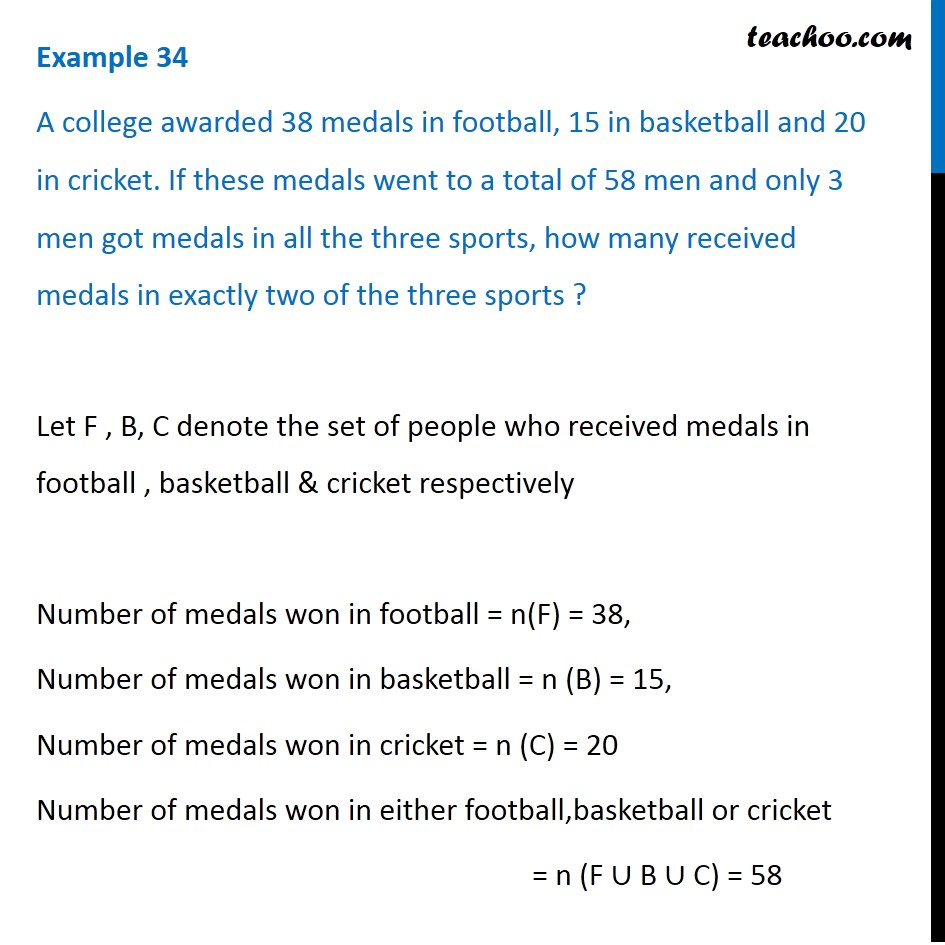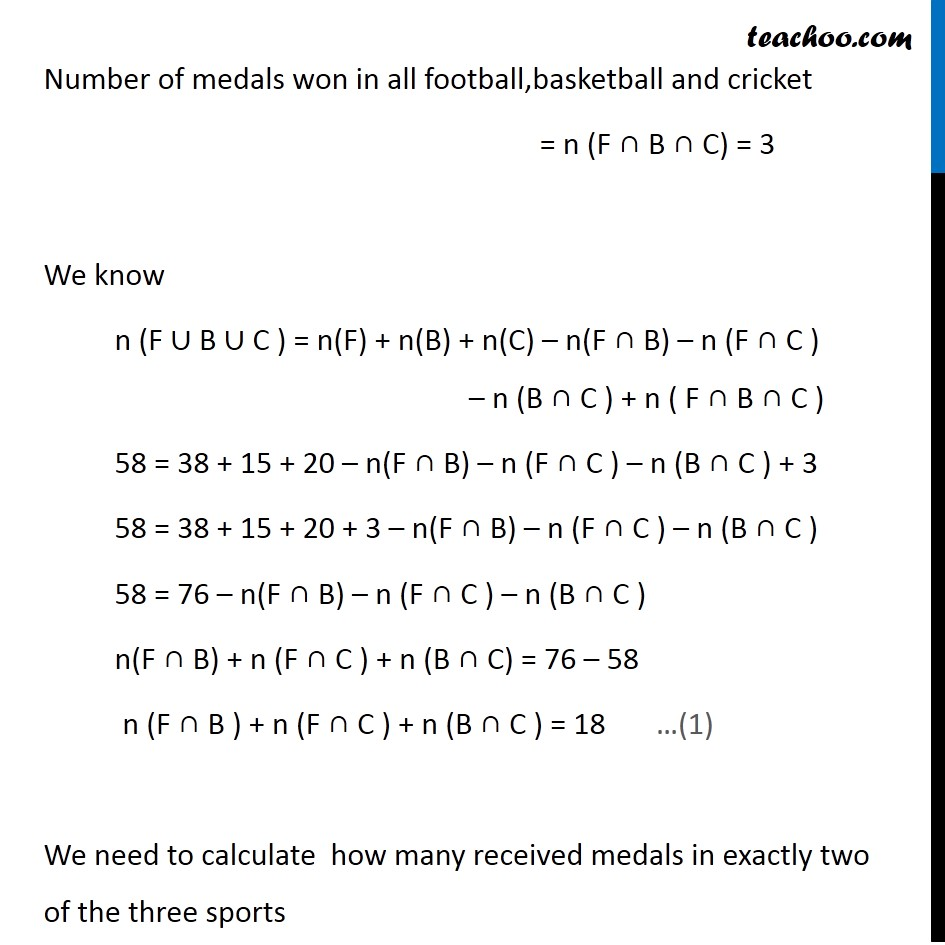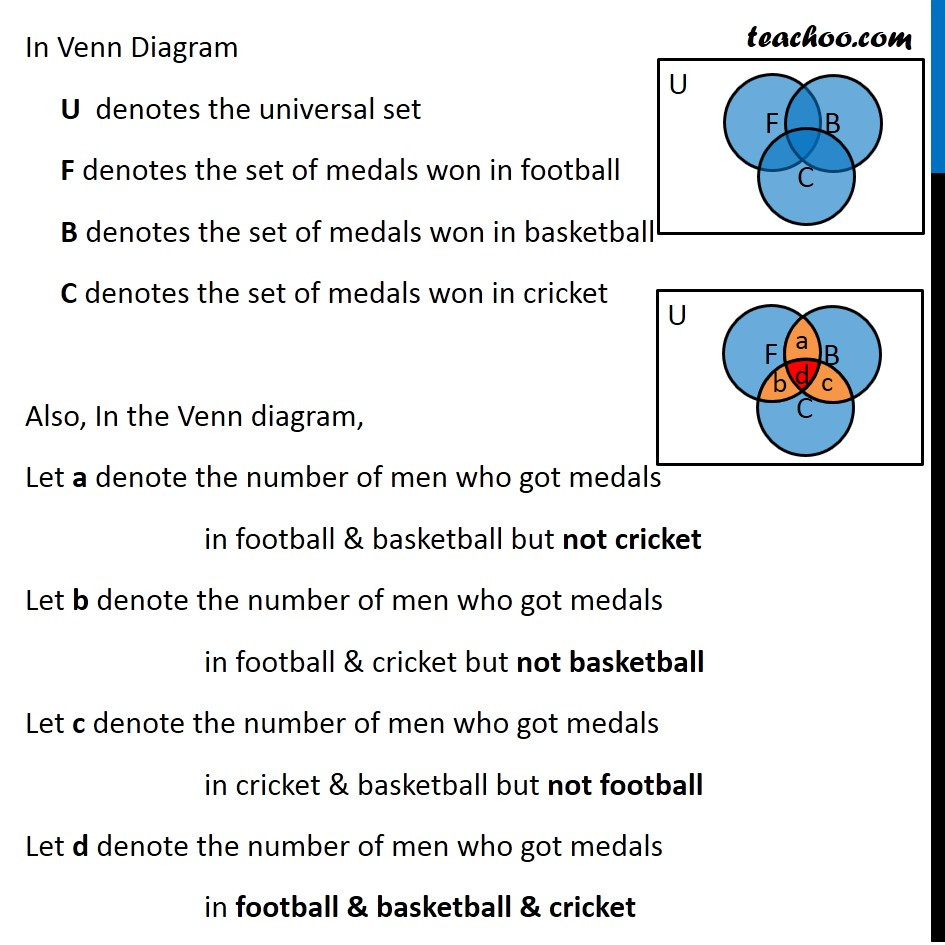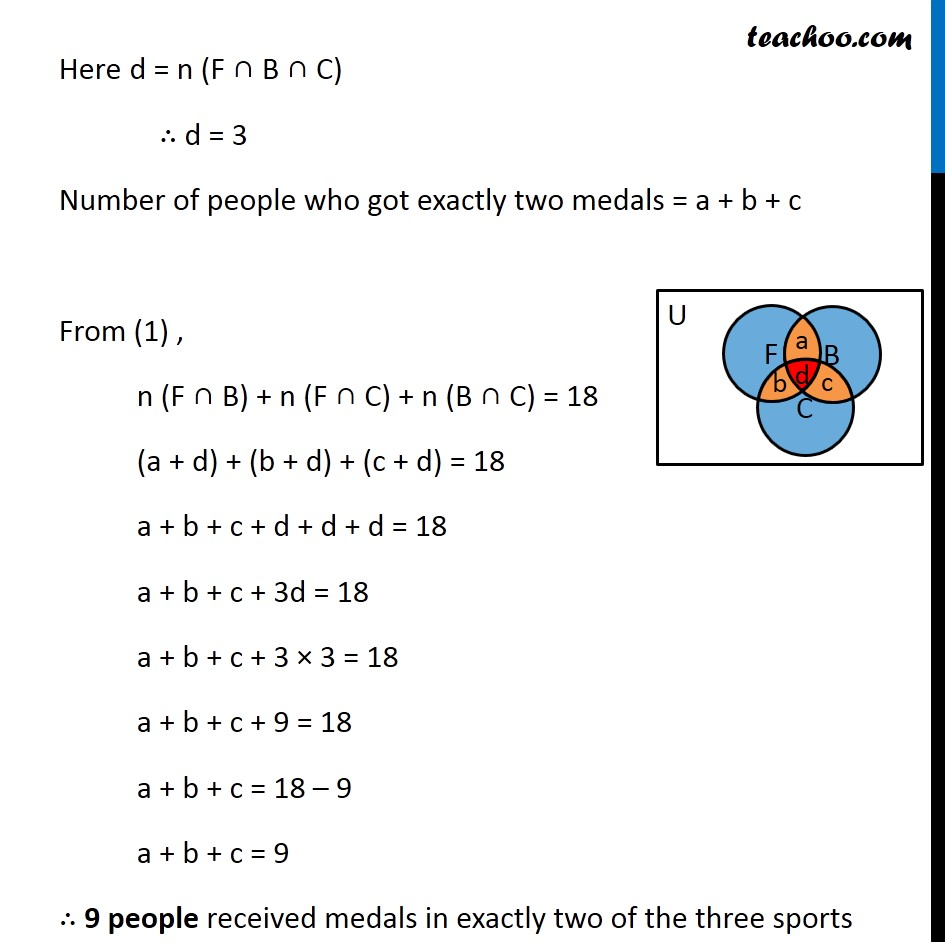1. Chapter 1 Class 11 Sets
2. Concept wise
3. Number of elements in set - 3 sets

Transcript

Example 34 A college awarded 38 medals in football, 15 in basketball and 20 in cricket. If these medals went to a total of 58 men and only 3 men got medals in all the three sports, how many received medals in exactly two of the three sports ? Let F , B, C denote the set of people who received medals in football , basketball & cricket respectively Number of medals won in football = n(F) = 38, Number of medals won in basketball = n (B) = 15, Number of medals won in cricket = n (C) = 20 Number of medals won in either football,basketball or cricket = n (F ∪ B ∪ C) = 58 Number of medals won in all football,basketball and cricket = n (F ∩ B ∩ C) = 3 We know n (F ∪ B ∪ C ) = n(F) + n(B) + n(C) – n(F ∩ B) – n (F ∩ C ) – n (B ∩ C ) + n ( F ∩ B ∩ C ) 58 = 38 + 15 + 20 – n(F ∩ B) – n (F ∩ C ) – n (B ∩ C ) + 3 58 = 38 + 15 + 20 + 3 – n(F ∩ B) – n (F ∩ C ) – n (B ∩ C ) 58 = 76 – n(F ∩ B) – n (F ∩ C ) – n (B ∩ C ) n(F ∩ B) + n (F ∩ C ) + n (B ∩ C) = 76 – 58 n (F ∩ B ) + n (F ∩ C ) + n (B ∩ C ) = 18 We need to calculate how many received medals in exactly two of the three sports In Venn Diagram U denotes the universal set F denotes the set of medals won in football B denotes the set of medals won in basketball C denotes the set of medals won in cricket Also, In the Venn diagram, Let a denote the number of men who got medals in football & basketball but not cricket Let b denote the number of men who got medals in football & cricket but not basketball Let c denote the number of men who got medals in cricket & basketball but not football Let d denote the number of men who got medals in football & basketball & cricket Here d = n (F ∩ B ∩ C) ∴ d = 3 Number of people who got exactly two medals = a + b + c From (1) , n (F ∩ B) + n (F ∩ C) + n (B ∩ C) = 18 (a + d) + (b + d) + (c + d) = 18 a + b + c + d + d + d = 18 a + b + c + 3d = 18 a + b + c + 3 × 3 = 18 a + b + c + 9 = 18 a + b + c = 18 – 9 a + b + c = 9 ∴ 9 people received medals in exactly two of the three sports

Number of elements in set - 3 sets

About the AuthorDavneet Singh
Davneet Singh is a graduate from Indian Institute of Technology, Kanpur. He has been teaching from the past 10 years. He provides courses for Maths and Science at Teachoo.